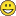# QlikView App Dev

Discussion Board for collaboration related to QlikView App Development.

cancel
Showing results for
Did you mean:Creator II

## How to use the max or maxstring function

Hi,

I have a field called FYQTR that will store data like FY2016-QR4, FY2016-QR3,FY2016-QR2, FY2016-QR1 and so each year. I have created 2 additional fields to store the numbers FY & QTR that will have 2016 and 4 respectively.

So, I have the following expression:

=Count({1<FY={2016}, QTR={">03"} >} AutoID)

Because I want to count the AutoID that appears when the FY is 2016 and QTR is 4.

I want to make this automatically, so I am trying to use the maxstring function but is not working at all.

I have tried:

=Count({1<MaxString(FYQTR)>} AutoID)
But does not work, any idea on how can I have the same functionality but automatically.

1 Solution

Accepted SolutionsMVP

May be try this:

=Count({1<FYQTR = {"\$(=MaxString(FYQTR))"}>} AutoID)

8 RepliesSpecialist

may be try with Dual() functionMVP

Perhaps This, This will give count of AutoId Where FY is 2016 and QTR is 4 and then FYQTR the value is maxstring of FYQTR

=Count({1<FY={2016}, FYQTR = {"=\$(MaxString(FYQTR))"}, QTR={'04'} >} AutoID)

Before develop something, think If placed (The Right information | To the right people | At the Right time | In the Right place | With the Right context)Creator II
Author

What would be the correct way to use the Dual() function in my script example?MVP

May be try this:

=Count({1<FYQTR = {"\$(=MaxString(FYQTR))"}>} AutoID)MVP

Anil the equal sign goes inside the dollar sign expansion (and not outside)

=Count({1<FY={2016}, FYQTR = {"=\$(MaxString(FYQTR))"}, QTR={'04'} >} AutoID)MVP

Yes Sunny, I thought this might work. Because i've tried same expression with diff fields using set operator. But it is given Good result. Anyway, Thanks for the solvation. Please correct me if i am wrong

If we created one variable like below

LET V1 = MaxString(FYQTR)

This case, We are not using the =  Symbol, right? From this below expression we are not using the = Symbol, Right

=Count({1<FY={2016}, FYQTR = {"=\$('\$(V1)')"}, QTR={'04'} >} AutoID)

Before develop something, think If placed (The Right information | To the right people | At the Right time | In the Right place | With the Right context)Creator II
Author

Thank you for the comments, everything worked properly with that.MVP

Awesome